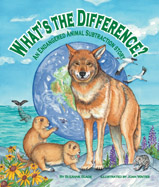Alignment to Standards for AK1 E&C-1. reasonable answers to real-life situations, paper/pencil computations, or calculator results by estimating 'how many' and 'how much' in a given set up to 20
1 E&C-3. solves problems involving recalling addition and subtraction facts 0-10 (M3.1.2)
1 F&R-3. adding and subtracting whole numbers to 20 using manipulatives to solve story problems (M4.1.4)
1 N-10. number theory by identifying fact families (M1.1.3)
1 N-6. mathematical operations by using objects, pictures, and problem situations to model addition and subtraction of whole numbers (M1.1.3)
1 N-7. identifying groups of objects as repeated addition or equal shares (M1.1.3)
2 E&C-4. solves problems involving recalling addition and subtraction facts to 20 (M3.1.2)
2 F&R-3. algebraic thinking by solving a problem with an unknown (e.g., 7 + ? = 10) (M4.1.4)
2 N-5. the processes of addition and subtraction of whole numbers and their relationships (M1.1.3)
2 N-6. number theory by skip counting by modeling or explaining the commutative and identity properties of addition (M1.1.7)
2 N-7. number theory (skip count by 2's, 5's, or 10's; add or subtract by 10; identify even or odd numbers) (M1.1.6)
2 N-8. number theory by modeling fact families (M1.1.3)
3 E&C-3 basic addition and subtraction facts, sums to 20, and corresponding subtraction facts
3 F&R-4 algebraic thinking by using an open number sentence (addition or subtraction) to solve for an unknown represented by a box or circle (e.g., 5+? =16, ?_7=4, 5+2=? ) (M4.1.4)
3 M1.1.3 processes of addition and subtraction, describing the relationship between the operations.
3 M1.1.7 Demonstrate the commutative and identity properties of addition.
3 M10.1.1 Apply mathematical skills and processes to literature.
3 M3.1.4 Model multiplication as repeated addition and grouping objects; model division as –sharing equally” and grouping objects.
3 M4.1.4 Complete open space sentences with missing numbers; use appropriate vocabulary including greater than, less than, and equal to; and use the correct symbols.
3 N-6 addition and subtraction of whole numbers and their relationships (M1.1.3)
3 N-8 number theory by modeling and explaining commutative property of addition (L) (M1.1.7)
3 SC1.1 sorting Alaskan plants and/or animals using physical characteristics (e.g., leaves, beaks
3 SC3.1 an understanding that all organisms are linked to each other and their physical environments through the transfer and transformation of matter and energy by identifying and sorting examples of living and non-living things in the local environment (L)
3 SC3.2 all organisms are linked to each other and their physical environments: a simple food chain of familiar plants and animals (L)
4 F&R-4 algebraic thinking by using an open number sentence (addition, subtraction, or multiplication) to solve for an unknown represented by a box or circle (e.g., 9 «? = 36, ?« 8=56, 3 « 6= ?) (M4.2.5)
4 SC2 understanding of the structure, function, behavior, development, life cycles, and diversity of living organisms.
4 SC2.2 an understanding of the structure, function, behavior, development, life cycles, and diversity of living organismscharacteristics and requirements of living things
4 SC3.1 an understanding that all organisms are linked to each other and their physical environments through the transfer and transformation of matter and energy by identifying examples of living and non-living things and the relationship between them (e.g., livi
4 SC3.2 all organisms are linked to each other and their physical environments: diagram how energy flows through a simple food chain
5 F&R-5 algebraic thinking by using an open number sentence (addition, subtraction, multiplication, or division) to solve for an unknown represented by a box or circle (e.g., 256 É? =8,?É8=56, 36É3=? ) (M4.2.5)
5 SC2.1 an understanding of the structure, function, behavior, development, life cycles, and diversity of living organisms by identifying and sorting animals into groups using basic external and internal features
5 SC3 understanding that all organisms are linked to each other and their physical environments through the transfer and transformation of matter and energy. (food webs, habitats)
5 SC3.2 all organisms are linked to each other and their physical environments: trace the source of the energy through a food chain
K E&C-1. comparing the number of objects in different sets using more, less, same
K E&C-3. solves problems involving adding and subtracting whole numbers up to ten using manipulatives (M3.1.3)
K F&R-4. adding or subtracting whole numbers to 10 using manipulatives to solve story problems (M4.1.4)
K N-10. model addition and subtraction of whole numbers (M1.1.3)
K N-9. mathematical operations by recognizing (+), (-), and (=) signs (M1.1.3)

Back to Standards Page